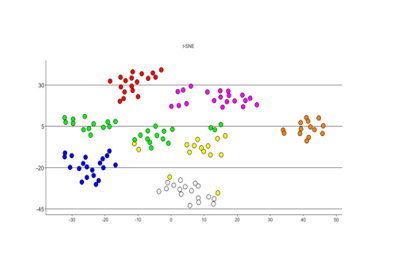# Introduction to t-SNE plot

Short introduction showing how Qlucore Omics Explorer provides easy to use t-SNE plots and the tools you need for single Cell analysis. The t-SNE plot is as easy to set-up and use as other plots. In addition to the t-SNE plot the program include tools such as pre-filtering and subsampling to support single cell data analysis.

Category: Introduction

Short introduction video

Watch here

Get started now with a free 10 days trial of Qlucore Omics Explorer!

Start here